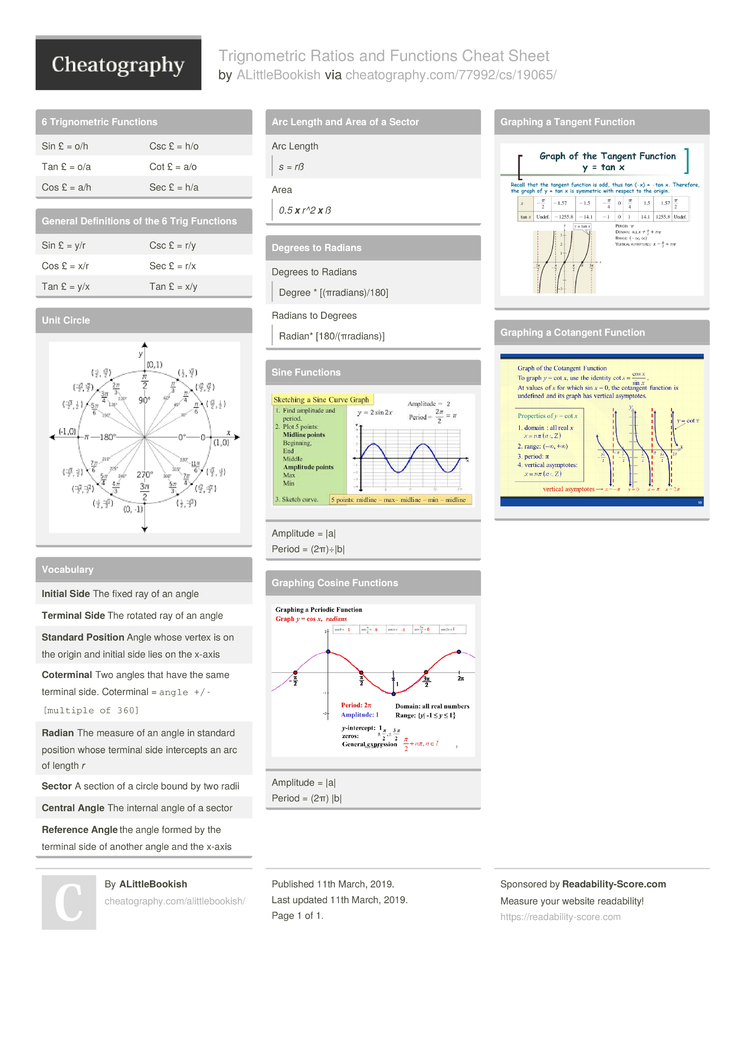# Trignometric Ratios and Functions Cheat Sheet by ALittleBookish

### 6 Trigno­metric Functions

 Sin £ = o/h Csc £ = h/o Tan £ = o/a Cot £ = a/o Cos £ = a/h Sec £ = h/a

### General Defini­tions of the 6 Trig Functions

 Sin £ = y/r Csc £ = r/y Cos £ = x/r Sec £ = r/x Tan £ = y/x Tan £ = x/y

### Unit Circle### Vocabulary

 Initial Side The fixed ray of an angle Terminal Side The rotated ray of an angle Standard Position Angle whose vertex is on the origin and initial side lies on the x-axis Cote­rmi­nal Two angles that have the same terminal side. Coterminal = `angle +/- [multiple of 360]` Radian The measure of an angle in standard position whose terminal side intercepts an arc of length r Sector A section of a circle bound by two radii Central Angle The internal angle of a sector Refe­rence Angle the angle formed by the terminal side of another angle and the x-axis

### Arc Length and Area of a Sector

 Arc Length s = rß Area0.5 x r^2 x ß

### Sine FunctionsAmplitude = |a|
Period = (2π)÷|b|

### Graphing Cosine FunctionsAmplitude = |a|
Period = (2π)➗|b|

### Graphing a Tangent Function### Graphing a Cotangent Function## Download the Trignometric Ratios and Functions Cheat Sheet1 Page
//media.cheatography.com/storage/thumb/alittlebookish_trignometric-ratios-and-functions.750.jpg

PDF (recommended)